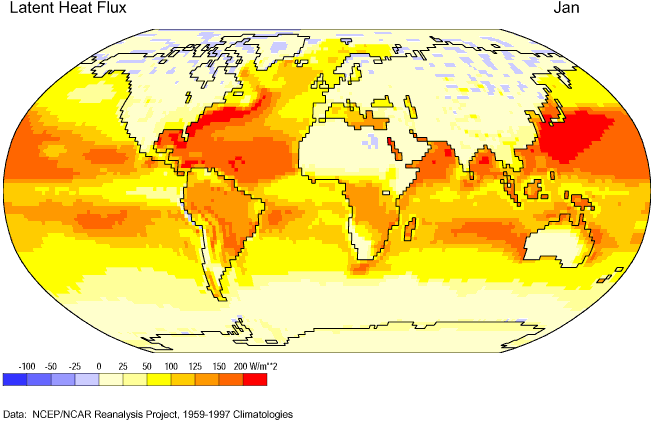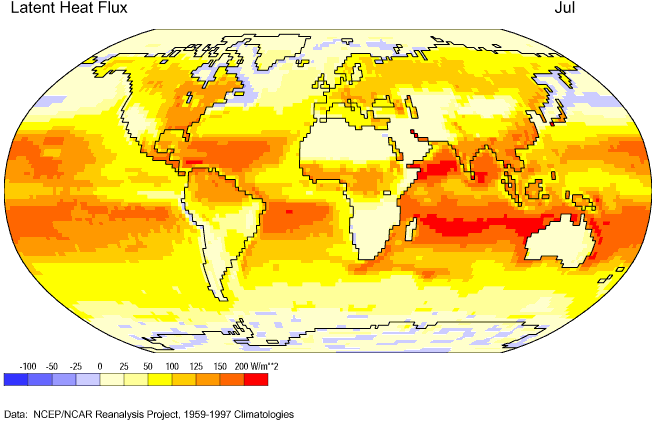PhysicalGeography.net | FUNDAMENTALS eBOOK

CHAPTER 6: Energy and Matter

# (c). Energy, Temperature, and Heat

So far we have learned that energy can take on many forms. One important form of energy, relative to life on Earth, is kinetic energy. Simply defined, kinetic energy is the energy of motion. The amount of kinetic energy that a body possesses is dependent on the speed of its motion and its mass. At the atomic scale, the kinetic energy of atoms and molecules is sometimes referred to as heat energy.

Kinetic energy is also related to the concept of temperature. Temperature is defined as the measure of the average speed of atoms and molecules. The higher the temperature, the faster these particles of matter move. At a temperature of -273.15° Celsius (absolute zero) all atomic motion stops. Heat is often defined as energy in the process of being transferred from one object to another because of difference in temperature between them. Heat is commonly transferred around our planet by the processes of conduction, convection, advection, and radiation.

Some other important definitions related to energy, temperature, and heat are:

Heat Capacity - is the amount of heat energy absorbed by a substance associated to its corresponding temperature increase.

Specific Heat - is equivalent to the heat capacity of a unit mass of a substance or the heat needed to raise the temperature of one gram (g) of a substance one degree Celsius. Water requires about 4 to 5 times more heat energy to raise its temperature when compared to an equal mass of most types of solid matter. This explains why water bodies heat more slowly than adjacent land surfaces.

Sensible Heat - is heat that we can sense. A thermometer can be used to measure this form of heat. Several different scales of measurement exist for measuring sensible heat. The most common are: Celsius scale, Fahrenheit scale, and the Kelvin scale.

Latent Heat - is the energy needed to change a substance to a higher state of matter. This same energy is released from the substance when the change of state (or phase) is reversed. The diagram below describes the various exchanges of heat involved with 1 gram of water.Figure 6c-1: Latent heat exchanges of energy involved with the phase changes of water.

Figures 6c-2 and 6c-3 show the net absorption and release of latent heat energy for the Earth's surface for January and July, respectively. The highest values of flux or flow occur near the subtropical oceans where high temperatures and a plentiful supply of water encourage the evaporation of water. Negative values of latent heat flux indicate a net release of latent energy back into the environment because of the condensation or freezing of water. Values of latent heat flux are generally low over landmasses because of a limited supply of water at the ground surface.Figure 6c-2: Mean January latent heat flux for the Earth's surface, 1959-1997. (Source of Original Modified Image: Climate Lab Section of the Environmental Change Research Group, Department of Geography, University of Oregon - Global Climate Animations).Figure 6c-3: Mean July latent heat flux for the Earth's surface, 1959-1997. (Source of Original Modified Image: Climate Lab Section of the Environmental Change Research Group, Department of Geography, University of Oregon - Global Climate Animations).

Study Guide